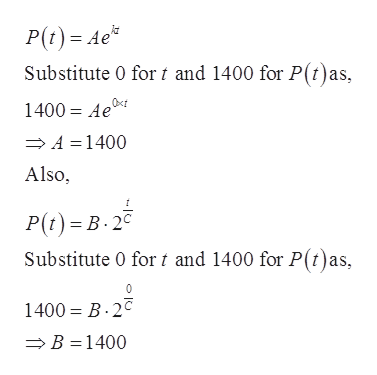# A culture contains 1400 bacteria initially and doubles every 50 minutes. Assuming that the rate of growth is proportional to the number of bacteria, find a function that models the number P(t) of bacteria after t minutes. P(t) = Aekt = B 2t/C , where A = k = B = C =Find the time T that it will take for the culture to reach 5800 bacteria? T = minutes

Question
44 views

A culture contains 1400 bacteria initially and doubles every 50 minutes. Assuming that the rate of growth is proportional to the number of bacteria, find a function that models the number P(t) of bacteria after t minutes.

P(t) = Aekt = B 2t/C , where
A =
k =
B =
C =

Find the time T that it will take for the culture to reach 5800 bacteria?

T = minutes

check_circle

Step 1

To determine a function P(t) that models the number of bacteria after t minutes.

Step 2

Given:

The initial number of bacteria is 1400 and the bacteria doubles every 50 minutes. The provided growth model is as given below:

Step 3

Consider that the initial number of bacteria is 1400 and the bacteria doubles ever...help_outlineImage TranscriptioncloseP(t) Ae* Substitute 0 for t and 1400 for P(t)as, 1400 Ae A 1400 Also, P(t) B. 2 Substitute 0 for t and 1400 for P(t)as, 1400 B 2C B 1400 fullscreen

### Want to see the full answer?

See Solution

#### Want to see this answer and more?

Solutions are written by subject experts who are available 24/7. Questions are typically answered within 1 hour.*

See Solution
*Response times may vary by subject and question.
Tagged in

### Functions